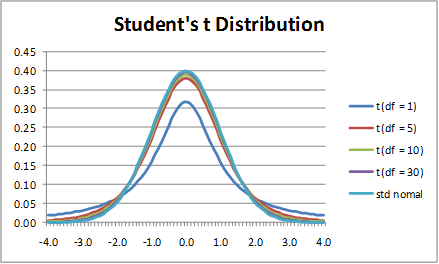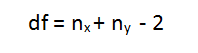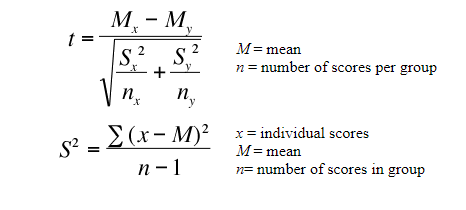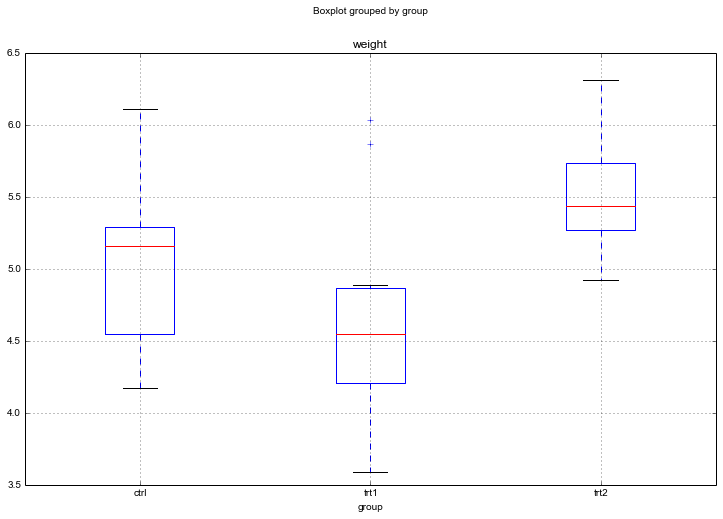# Python Code for TTEST and ANOVA

## TTEST

#### What is t-score?

The t score is a ratio between the difference between two groups and the difference within the groups.

#### Types of t-tests?

There are three main types of t-test:
1. An Independent Samples t-test compares the means for two groups.
2. A Paired sample t-test compares means from the same group at different times (say, one year apart).
3. A One sample t-test tests the mean of a single group against a known mean.

##two sample TTESTimport numpy as np
from scipy import stats

## Define 2 random distributions
#Sample Size
N = 10
#Gaussian distributed data with mean = 2 and var = 1
a = np.random.randn(N) + 2
#Gaussian distributed data with with mean = 0 and var = 1
b = np.random.randn(N)

## Calculate the Standard Deviation
#Calculate the variance to get the standard deviation

#For unbiased max likelihood estimate we have to divide the var by N-1, and therefore the parameter ddof = 1
var_a = a.var(ddof=1)
var_b = b.var(ddof=1)

#std deviation
s = np.sqrt((var_a + var_b)/2)
s

## Calculate the t-statistics
t = (a.mean() – b.mean())/(s*np.sqrt(2/N))

## Compare with the critical t-value
#Degrees of freedom
df = 2*N – 2

#p-value after comparison with the t
p = 1 – stats.t.cdf(t,df=df)

print(“t = ” + str(t))
print(“p = ” + str(2*p))
#Note that we multiply the p value by 2 because its a twp tail t-test
### You can see that after comparing the t statistic with the critical t value (computed internally) we get a good p value of 0.0005 and thus we reject the null hypothesis and thus it proves that the mean of the two distributions are different and statistically significant.

## Cross Checking with the internal scipy function
t2, p2 = stats.ttest_ind(a,b)
print(“t = ” + str(t2))
print(“p = ” + str(2*p2))

## One Way ANOVA

import pandas as pd
datafile=“PlantGrowth.csv”
data = pd.read_csv(datafile)
#Create a boxplot
data.boxplot(‘weight’, by=‘group’, figsize=(12, 8))
ctrl = data[‘weight’][data.group == ‘ctrl’]
grps = pd.unique(data.group.values)
d_data = {grp:data[‘weight’][data.group == grp] for grp in grps}
k = len(pd.unique(data.group))  # number of conditions
N = len(data.values)  # conditions times participants
n = data.groupby(‘group’).size() #Participants in each conditionJudging by the Boxplot there are differences in the dried weight for the two treatments. However, easy to visually determine whether the treatments are different to the control group.

## Using SciPy$SSbetween = \frac{\sum(\sum k_i) ^2} {n} - \frac{T^2}{N}$$SSwithin = \sum Y^2 - \frac{\sum (\sum a_i)^2}{n}$$SStotal = \sum Y^2 - \frac{T^2}{N}$
from scipy import stats
F, p = stats.f_oneway(d_data[‘ctrl’], d_data[‘trt1’], d_data[‘trt2’])
DFbetween = k 1
DFwithin = N k
DFtotal = N 1
SSbetween = (sum(data.groupby(‘group’).sum()[‘weight’]**2)/n) \
(data[‘weight’].sum()**2)/N
sum_y_squared = sum([value**2 for value in data[‘weight’].values])
SSwithin = sum_y_squared sum(data.groupby(‘group’).sum()[‘weight’]**2)/n
SStotal = sum_y_squared (data[‘weight’].sum()**2)/N
MSbetween = SSbetween/DFbetween
MSwithin = SSwithin/DFwithin
F = MSbetween/MSwithin
p = stats.f.sf(F, DFbetween, DFwithin)
eta_sqrd = SSbetween/SStotal  # effect size
om_sqrd = (SSbetween (DFbetween * MSwithin))/(SStotal + MSwithin)  # omega effect size (less biased)

## Using STATSMODELS

import statsmodels.api as sm
from statsmodels.formula.api import ols
mod = ols(‘weight ~ group’,
data=data).fit()

aov_table = sm.stats.anova_lm(mod, typ=2)
print aov_table

#### Output table:

sum_sq df F PR(>F)
group 3.76634 2 4.846088 0.01591
Residual 10.49209 27

# calculate effect size

esq_sm = aov_table[‘sum_sq’]/(aov_table[‘sum_sq’]+aov_table[‘sum_sq’])

## Using pyvttbl anova1way

from pyvttbl import DataFrame
df=DataFrame()
df.read_tbl(datafile)
aov_pyvttbl = df.anova1way(‘weight’, ‘group’)
print aov_pyvttbl
Anova: Single Factor on weight
SUMMARY
Groups   Count    Sum     Average   Variance
============================================
ctrl        10   50.320     5.032      0.340
trt1        10   46.610     4.661      0.630
trt2        10   55.260     5.526      0.196
O’BRIEN TEST FOR HOMOGENEITY OF VARIANCE
Source of Variation    SS     df    MS       F     P-value   eta^2   Obs. power
===============================================================================
Treatments            0.977    2   0.489   1.593     0.222   0.106        0.306
Error                 8.281   27   0.307
===============================================================================
Total                 9.259   29
ANOVA
Source of Variation     SS     df    MS       F     P-value   eta^2   Obs. power
================================================================================
Treatments             3.766    2   1.883   4.846     0.016   0.264        0.661
Error                 10.492   27   0.389
================================================================================
Total                 14.258   29
POSTHOC MULTIPLE COMPARISONS
Tukey HSD: Table of q-statistics
ctrl     trt1       trt2
=================================
ctrl   0      1.882 ns   2.506 ns
trt1          0          4.388 *
trt2                     0
=================================
+ p < .10 (q-critical[3, 27] = 3.0301664694)
* p < .05 (q-critical[3, 27] = 3.50576984879)
** p < .01 (q-critical[3, 27] = 4.49413305084)